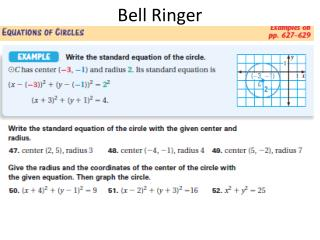DownloadDownload PresentationBell Ringer

# Bell Ringer

Télécharger la présentation## Bell Ringer

- - - - - - - - - - - - - - - - - - - - - - - - - - - E N D - - - - - - - - - - - - - - - - - - - - - - - - - - -
##### Presentation Transcript

1. Bell Ringer

2. If-Then Statements and Deductive Reasoning

3. An if-then statement has two parts. The “if” part contains the hypothesis. The “then” part contains the conclusion.

4. Example 1 SOLUTION I pass the driving test is the hypothesis. Identify the Hypothesis and Conclusion Identify the hypothesis and the conclusion of the if-then statement. If I pass the driving test, then I will get my driver’s license. I will get my driver’s license is the conclusion.

5. Example 2 SOLUTION Write If-Then Statements Rewrite the statement as an if-then statement. a. Every game on my computer is fun to play. b. I will buy the CD if it costs less than \$15. a. If a game is on my computer, then it is fun to play. b. If the CD costs less than \$15, then I will buy it.

6. Deductive Reasoning uses facts, definitions, accepted properties, and the laws of logic to make a logical argument.

7. Example 3 What can you conclude from the following true statements? If you wash the cotton T-shirt in hot water, then it will shrink. You wash the cotton T-shirt in hot water. SOLUTION The hypothesis (you wash the cotton T-shirt in hot water) of a true if-then statement is true. By the Law of Detachment, the conclusion must be true. Use the Law of Detachment You can conclude that the cotton T-shirt shrinks. ANSWER

8. Now You Try  1. All teachers at East High School have taught for at least 5 years. If a teacher works at East High School, then ANSWER he or she has taught for at least 5 years. 2. An angle is obtuse if its measure is 170°. If the measure of an angle is 170°, then ANSWER the angle is obtuse. If-Then Statements and the Law of Detachment Rewrite the statement as an if-then statement. Then underline the hypothesis and circle the conclusion.

9. Checkpoint 3. If xhas a value of 7, then 2x –3 has a value of 11. The value of xis 7. 2x – 3has a value of 11. ANSWER 4. If you study at least two hours for the test, then you will pass the test. You study three hours for the test. You will pass the test. ANSWER If-Then Statements and the Law of Detachment Now You Try  What can you conclude from the given true statements?

10. Use the Law of Detachment Example 4 SOLUTION Argument 2 is correct. The hypothesis (two angles are vertical angles) is true, which implies that the conclusion (they are congruent) is true. Which argument is correct? Explain your reasoning. Argument 1: If two angles are vertical angles, then they are congruent. 1and 2 are congruent. So, 1 and 2 are vertical angles. Argument 2: If two angles are vertical angles, then they are congruent. 1 and 2 are vertical angles. So, 1 and 2 are congruent. You can use the following counterexample to show that Argument 1 is false. In the diagram at the right, 1 2, but they are not vertical angles.

11. Checkpoint ANSWER Argument 1 is correct. In Argument 1, the hypothesis is true, which implies that the conclusion is true. The following counterexample shows that Argument 2 is false: Two nonadjacent angles with measures 40° and 140° are supplementary, but they do not form a linear pair. Now You Try  Use the Law of Detachment 5. Which argument is correct? Explain your reasoning. Argument 1: If two angles from a linear pair, then they are supplementary. 3and 4 from a linear pair. So, 3 and 4 are supplementary. Argument 2: If two angles from a linear pair, then they are supplementary. 3 and 4 are supplementary. So, 3 and 4 from a linear pair.

12. Example 5 SOLUTION Use the Law of Syllogism. Use the Law of Syllogism Write the statement that follows from the pair of true statements. If the daily high temperature is 32°F or less, then the water in the pipe is frozen. If the water in the pipe is frozen, then the pipe will break. If the daily high temperature is 32°F or less, then the pipe will break.

13. Checkpoint 6. Write the statement that follows from the pair of true statements. If the ball is thrown at the window, it will hit the window. If the ball hits the window, then the window will break. Now You Try  Use the Law of Syllogism If the ball is thrown at the window, then the window will break. ANSWER

14. Page 85

15. Page 85

16. Complete Pages 85-87 #s 2-26 even only